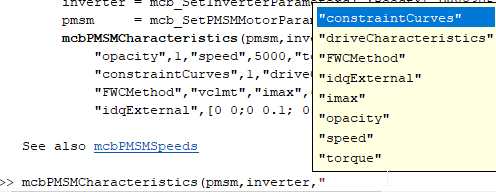# PMSM Drive Characteristics and Constraint Curves

This example uses Motor Control Blockset™ to show how to use the PMSM characteristic plotting and PMSM milestone speed identification functions to obtain a control trajectory.

### Required MathWorks® Products

• Motor Control Blockset

### PMSM Drive Characteristics and Constraint Curves

The permanent magnet synchronous motors (PMSMs) come in different configurations.

• When the permanent magnets (PMs) are mounted on the surface of the rotor, the motor is called a surface-mounted PMSM (SPMSM).

• When the PMs are embedded inside the rotor below the surface, the motor is called an interior PMSM (IPMSM).

Various parameters affect whether you select an SPMSM or IPMSM for a given application.

In the $\mathit{d}$-$\mathit{q}$ rotor reference frame for PMSMs, the $\mathit{d}$-axis is the axis parallel to the rotor's magnetic orientation and the $\mathit{q}$-axis is the perpendicular axis, leading the $\mathit{d}$-axis. An SPMSM has equal $\mathit{d}$-axis and $\mathit{q}$-axis inductances. An IPMSM has a higher $\mathit{q}$-axis inductance than its $\mathit{d}$-axis inductance. This helps the IPMSM to utilize the reluctance torque in addition to the magnetic torque.

The corner speed of a PMSM is the speed at which the torque-vs-speed (drive characteristics) curve of the PMSM changes shape (has a corner) for a given current limit. When you plot the drive characteristics for rated torque (at rated current), the corner speed is also known as the rated speed or base speed.You draw the constraint curves for a PMSM to understand the possible operational area and boundaries.

• Maximum torque per ampere (MTPA) trajectory of an IPMSM provides the highest torque for the allowed stator current.

• Maximum torque per voltage (MTPV) trajectory of an IPMSM provides the highest torque while satisfying the stator voltage constraint.

• Voltage limit curve (ellipse for an IPMSM or circle for SPMSM) is centered around a point that is called the characteristic current. This curve shrinks with increasing rotor speed.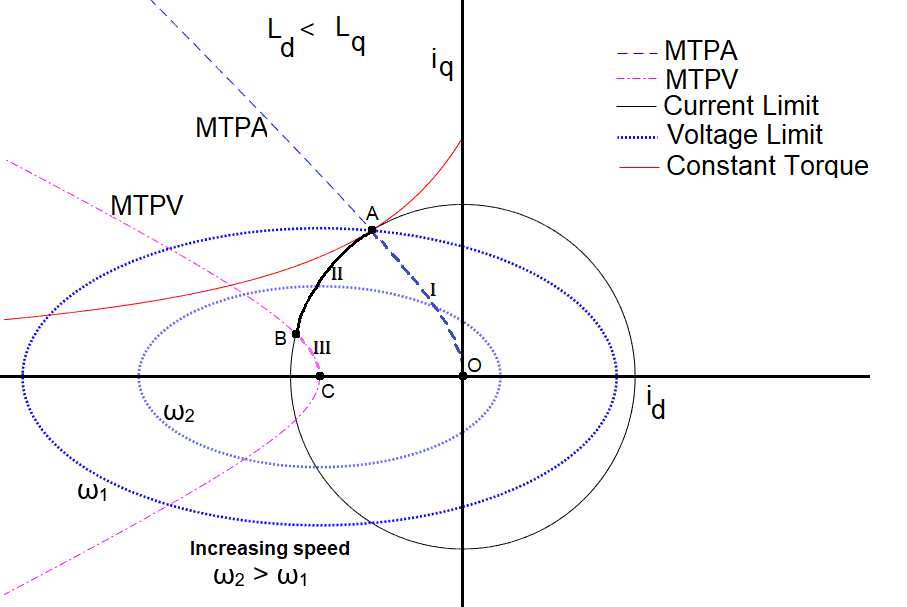These figures show three segments corresponding to the motor operation. The motor operates in each segment as follows.

#### Segment I

• To begin the operation, the motor increases the torque from zero to the rated torque.

• The operating point shifts from point O to A along the MTPA trajectory (line segment OA). This corresponds to the ${\mathit{i}}_{\mathit{d}}$ and ${\mathit{i}}_{\mathit{q}}$ values at the tip of MTPA.

• The motor speed ${\omega }_{1}$, at which the voltage limit ellipse touches the MTPA curve and the current limit circle, is the rated speed. This is the first milestone speed of the characteristic curve.

#### Segment II

• At the operating point A, the control reaches the maximum possible current, and the field weakening strategy begins.

• The current trajectory moves along the current limit circle while maintaining the current constraint from point A to B (line segment AB).

• The operating point B is the speed at which the voltage ellipse touches the current limit circle and the MTPV curve. This is the second milestone speed of the characteristic curve.

#### Segment III

• Beyond the point B, the voltage ellipse shrinks further and the MTPV trajectory provides the optimal torque until the maximum speed is reached at the operating point C (line segment BC).

• The theoretical maximum speed for a friction-free motor with MTPV is infinite. Practically, the frictional components in the motor limit the maximum speed to a finite value. In Motor Control Blockset™ software, the maximum speed, including the friction, is when the frictional torque balances the motor torque produced at that speed. The practical speed limit of the motor is usually lower than this maximum speed value. Several factors regulate this limit, including mechanical stability, lower efficiency at higher speeds, and so on.

• The calculated maximum speed is the third milestone speed. In some motor configurations, MTPV is not possible. In such cases, the frictional torque limits the operating point B, and there is no operating point C. Such motors have two milestone speeds.

Additionally, note that in these figures:

• The drive characteristic curves show the operating points O, A, and B. These curves do not show the operating point C because it is far away from the remaining points.

• The operating point A is also at the rated speed ${\omega }_{1}$. The second speed ${\omega }_{2}$ for the curve is higher than rated speed, but lower than the speed at operating point B. The speed at the operating point B is also the speed at which the current trajectory changes direction from following the current limit circle to following the MTPV trajectory.

• The drive characteristics do not show segment I because the motor maintains torque at maximum value, and the ${\mathit{i}}_{\mathit{d}}$ and ${\mathit{i}}_{\mathit{q}}$ values remain at values corresponding to the rated torque.

This table shows the functions used in this example.The `pmsm` and `inverter` arguments are structures. This table shows the fields in each structure.### Maximum and Milestone Speeds of PMSM

To get all the milestone speeds of the PMSM, use the `mcbPMSMSpeeds` function. The operable speeds are different for different field weakening control (FWC) strategies. The default FWC method is set to voltage current limited maximum torque (VCLMT) control, also called optimal current vector control. The function returns the speed values in rpm.

Set the fields for the `pmsm` and `inverter` structures and calculate the milestone speeds.

```%inverter = mcb_SetInverterParameters( 'BoostXL-DRV8305'); % Use an inverter structure from a template inverter.V_dc=24; pmsm = mcb_SetPMSMMotorParameters('BLY171D'); milestone_speeds=mcbPMSMSpeeds(pmsm,inverter); disp(milestone_speeds);```
``` 5393 9373 ```
```pmsm.I_rated=8;% Assuming a higher I_rated to be able to get MTPV trajectory [milestone_speeds]=mcbPMSMSpeeds(pmsm,inverter); disp(milestone_speeds); ```
``` 2342 2958 24369 ```

### Speed Milestones of PMSM with Different FW Control Methods

Calculate speed milestones for different field weakening methods. When you set the `verbose` option to `1`, each command displays the milestone speeds with the description.

Set the fields for the `pmsm` and `inverter` structures and calculate the milestone speeds.

```inverter = mcb_SetInverterParameters( 'BoostXL-DRV8305'); pmsm = mcb_SetPMSMMotorParameters('BLY171D'); verbose=0; % setting the verbose=1 will also print the messages```
```milestone_speeds=mcbPMSMSpeeds(pmsm,inverter,'verbose',verbose,'FWCMethod','vclmt'); disp(milestone_speeds);```
``` 5393 9373 ```
```milestone_speeds=mcbPMSMSpeeds(pmsm,inverter,'verbose',verbose,'FWCMethod','cvcp'); disp(milestone_speeds);```
``` 5393 7008 8361 ```
```milestone_speeds=mcbPMSMSpeeds(pmsm,inverter,'verbose',verbose,'FWCMethod','cccp'); disp(milestone_speeds);```
``` 5393 7008 ```
```milestone_speeds=mcbPMSMSpeeds(pmsm,inverter,'verbose',verbose,'FWCMethod','none'); % no id control, id is always set to 0. disp(milestone_speeds);```
``` 5393 6226 ```

### ${\mathit{i}}_{\mathit{d}}$ and ${\mathit{i}}_{\mathit{q}}$ at Different Milestone Speeds for Different FW Control Methods

Calculate ${\mathit{i}}_{\mathit{d}}$ and ${\mathit{i}}_{\mathit{q}}$ values at the speed milestones for different field weakening methods. Each command outputs the array with columns for each milestone speed, and each row of the column provides ${\mathit{i}}_{\mathit{d}}$, ${\mathit{i}}_{\mathit{q}}$, and speed (in electrical rad/s).

Set the fields for the `pmsm` and `inverter` structures and calculate currents and milestone speeds.

```inverter = mcb_SetInverterParameters( 'BoostXL-DRV8305'); pmsm = mcb_SetPMSMMotorParameters('BLY171D');```
```[milestone_speeds]=mcbPMSMSpeeds(pmsm,inverter,'FWCMethod','vclmt','outputAll',0); [outputArray]=mcbPMSMSpeeds(pmsm,inverter,'FWCMethod','vclmt','outputAll',1); disp(outputArray);```
``` 1.0e+03 * 0 -0.0018 0.0018 0.0004 2.2591 3.9262 ```
`disp(round([milestone_speeds(1) outputArray(3,1)*(60/(2*pi))/pmsm.p; milestone_speeds(2) outputArray(3,2)*(60/(2*pi))/pmsm.p])); % comparing the corner rpm & max rpm, and the outputArray's corresponding field.`
``` 5393 5393 9373 9373 ```
```outputArray=mcbPMSMSpeeds(pmsm,inverter,'FWCMethod','cvcp','outputAll',1); disp(outputArray);```
``` 1.0e+03 * 0 -0.0011 -0.0018 0.0018 0.0014 0.0003 2.2591 2.9355 3.5023 ```
```outputArray=mcbPMSMSpeeds(pmsm,inverter,'FWCMethod','cccp','outputAll',1); disp(outputArray);```
``` 1.0e+03 * 0 -0.0011 0.0018 0.0014 2.2591 2.9355 ```
```outputArray=mcbPMSMSpeeds(pmsm,inverter,'FWCMethod','none','outputAll',1); disp(outputArray);```
``` 1.0e+03 * 0 0 0.0018 0.0002 2.2591 2.6081 ```

### Plot Drive Characteristics

The drive characteristics display the torque-vs-speed, power-vs-speed, and current-vs-speed characteristic plots of a motor under the given operating constraints. Use the `mcbPMSMCharacteristics` function to plot the drive characteristics.

Plot the drive characteristics of the PMSM under different field weakening control methods. You can also plot the constraint curves simultaneously, in which case, you also plot the current trajectory (to get maximum torque for a given speed) for the chosen drive characteristics. When you set the `driveCharacteristics` option to `2`, the function plots an additional figure in the ${\mathit{v}}_{\mathit{d}}$-${\mathit{v}}_{\mathit{q}}$ space with the voltage constraint curve and the voltage trajectory. The default field weakening control is set to VCLMT.

Set the fields for the `pmsm` and `inverter` structures and plot the characteristics.

```inverter = mcb_SetInverterParameters( 'BoostXL-DRV8305'); pmsm = mcb_SetPMSMMotorParameters('BLY171D'); mcbPMSMCharacteristics(pmsm,inverter,'driveCharacteristics',2,'constraintCurves',1,'FWCMethod','vclmt')````mcbPMSMCharacteristics(pmsm,inverter,'driveCharacteristics',1,'constraintCurves',1,'FWCMethod','cvcp')``mcbPMSMCharacteristics(pmsm,inverter,'driveCharacteristics',1,'constraintCurves',1,'FWCMethod','cccp')``mcbPMSMCharacteristics(pmsm,inverter,'driveCharacteristics',1,'constraintCurves',1,'FWCMethod','none')`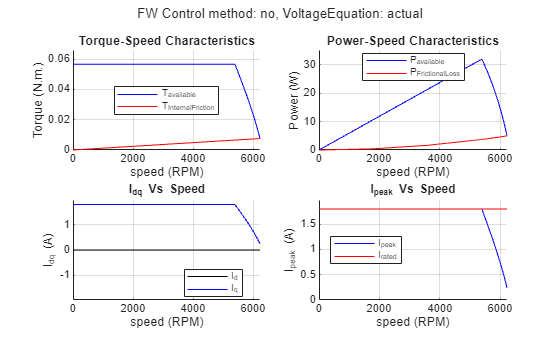### Plot Drive Characteristics for ${\mathit{I}}_{\mathrm{max}}$

When the motor operates continuously supporting a constant load torque, that condition is the rated operating condition, and the operating current is the rated current. The short-term rating of the motor is higher than the continuous-current rating.

For this example, `pmsm.I_rated` is set to 1.8 A and `pmsm.I_max` is set to 8 A. You can see the comparison between the drive characteristics and use this to determine the short-term operating characteristics.

```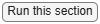inverter = mcb_SetInverterParameters( 'BoostXL-DRV8305'); pmsm = mcb_SetPMSMMotorParameters('BLY171D'); mcbPMSMCharacteristics(pmsm,inverter,"FWCMethod","vclmt","driveCharacteristics",1,"imax",8,"constraintCurves",0)```Next, assume a high continuous rating to let the motor run with MTPV under rated conditions. The maximum current ${\mathit{I}}_{\mathrm{max}}$ also operates the motor under the MTPV condition, resulting in no change in the maximum speed.

```pmsm.I_rated=6; mcbPMSMCharacteristics(pmsm,inverter,"FWCMethod","vclmt","driveCharacteristics",1,"imax",10,"constraintCurves",0)```When you specify the field weakening method as `none`, you might not see a change in maximum speed for the original motor (unchanged rated current) even with higher current limit, but only the peak torque and power changes.

```pmsm.I_rated=1.8; % setting the rated current to the normal (low) value) mcbPMSMCharacteristics(pmsm,inverter,"FWCMethod","cvcp","driveCharacteristics",1,"imax",8,"constraintCurves",0)````mcbPMSMCharacteristics(pmsm,inverter,"FWCMethod","cccp","driveCharacteristics",1,"imax",8,"constraintCurves",0)``mcbPMSMCharacteristics(pmsm,inverter,"FWCMethod","none","driveCharacteristics",1,"imax",8,"constraintCurves",0)`### Customize Drive Characteristics

Customize the plots using the optional name-value arguments for the characteristics function.

The `pmsm` structure expects values for the `p`, `Rs`, `Ld`, `Lq`, `FluxPM`, `B` and `I_rated` fields. The `inverter` structure expects a value for the `V_dc` field. Set the field values and plot the characteristics.

```inverter.V_dc=24; pmsm.p=4; pmsm.Rs=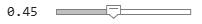0.45; pmsm.Ld=1e-3; pmsm.Lq=pmsm.Ld*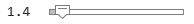1.4; pmsm.FluxPM=5.2e-3; pmsm.B=1.16e-5; pmsm.I_rated=2; w_rpm=7000; T_load=0.01; FWCMethod=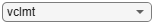"vclmt"; mcbPMSMCharacteristics(pmsm,inverter,'speed',w_rpm,'torque',T_load,'FWCMethod',FWCMethod, 'constraintCurves',0,'driveCharacteristics',1);```### Plot Constraint Curves in ${\mathit{i}}_{\mathit{d}}$-${\mathit{i}}_{\mathit{q}}$ Space

You can plot the constraint curves for PMSM in the ${\mathit{i}}_{\mathit{d}}$-${\mathit{i}}_{\mathit{q}}$ space using the `mcbPMSMCharacteristics` function.

Set the fields for the `pmsm` and `inverter` structures and plot the characteristics.

```inverter = mcb_SetInverterParameters( 'BoostXL-DRV8305'); pmsm = mcb_SetPMSMMotorParameters('BLY171D'); pmsm.Rs=0.1; pmsm.Lq=pmsm.Ld*1.8; w_rpm=5000; T_load=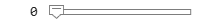0; mcbPMSMCharacteristics(pmsm,inverter,'speed',w_rpm,'torque',T_load); legend("Position", [-0.044093,0.16512,0.60536,0.2]) xlim([-14.7 2.1]) ylim([-12.5 7.6])```### Plot Constraint Curves at Maximum Speed

The constraint curves provide a secondary check for whether the calculated speeds are correct. When you plot the constraint curves at maximum speed, the plot shows three intersecting curves: current limit circle, voltage limit curve, and constant torque curve.

```inverter = mcb_SetInverterParameters( 'BoostXL-DRV8305'); pmsm = mcb_SetPMSMMotorParameters('BLY171D'); pmsm.Rs=0.1; pmsm.Lq=pmsm.Ld*1.8; milestone_speeds=mcbPMSMSpeeds(pmsm,inverter); mcbPMSMCharacteristics(pmsm,inverter,'speed',milestone_speeds(end),torque=0) xlim([-9.1 2.2]) ylim([-5.9 6.0]) legend("Position",[0.57813,0.64246,0.59643,0.2869])```### Plot Constraint Curves at Milestone Speeds

Instead of plotting the constraint curves for all the speed milestones in different figures, you can plot them together in the same characteristic plot. In addition to the intersecting curves at maximum speed, notice that at the corner speed, the MTPA curve, the current limit circle, and the voltage constraint curve intersect.

```inverter = mcb_SetInverterParameters( 'BoostXL-DRV8305'); pmsm = mcb_SetPMSMMotorParameters('BLY171D'); pmsm.Lq=pmsm.Ld*1.8; pmsm.Rs=0.1; [milestone_speeds]=mcbPMSMSpeeds(pmsm,inverter,'constraintCurves',1); legend("Position",[0.61205,0.66151,0.375,0.22976]) ax = gca; chart = ax.Children(3); datatip(chart,-3.099,-3.697,"Location","southwest"); chart = ax.Children(9); datatip(chart,-2.764,3.847,"Location","northwest"); xlim([-15.0 1.8]) ylim([-12.2 12.3])```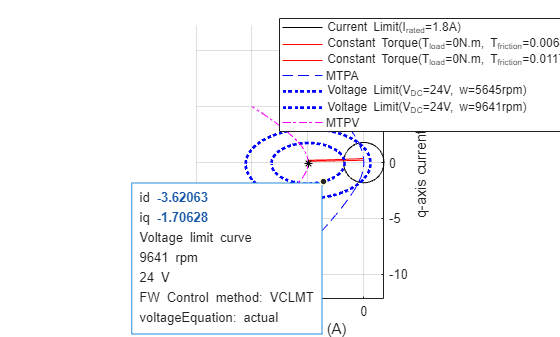`disp(milestone_speeds);`
``` 5645 9641 ```

### Plot Constraint Curves with Different Speeds in Same Figure

Plot the constraint curves of the motor at different speeds in the same figure.

Use the `opacity` option to set the opacity of the presently plotted constraint curve.

• When you set `opacity` to `1` (default), the function creates a new figure window for plotting.

• When you set `opacity` to a value less than 1 (but more than 0), the function plots the voltage constraint, MTPA, and MTPV curves with changing opacity.

The `opacity` option is useful when you want to identify changes and track trends in these curves.

```inverter = mcb_SetInverterParameters( 'BoostXL-DRV8305'); pmsm = mcb_SetPMSMMotorParameters('BLY171D'); pmsm.Rs=0.1; pmsm.Lq=pmsm.Ld*1.8; mcbPMSMCharacteristics(pmsm,inverter,'speed',4000,'torque',0,'opacity',1) mcbPMSMCharacteristics(pmsm,inverter,'speed',5000,'opacity',0.8) mcbPMSMCharacteristics(pmsm,inverter,'speed',6000,'opacity',0.7) mcbPMSMCharacteristics(pmsm,inverter,'speed',7000,'opacity',0.6) mcbPMSMCharacteristics(pmsm,inverter,'speed',8000,'opacity',0.5) mcbPMSMCharacteristics(pmsm,inverter,'speed',9000,'opacity',0.4) mcbPMSMCharacteristics(pmsm,inverter,'speed',10000,'opacity',0.3) mcbPMSMCharacteristics(pmsm,inverter,'speed',11000,'opacity',0.2) mcbPMSMCharacteristics(pmsm,inverter,'speed',12000,'opacity',0.1) xlim([-7.61 1.23]) ylim([-1.55 9.05]) ax2 = gca; chart2 = ax2.Children(3); datatip(chart2,-7.541,-0.4029,"Location","southwest"); chart2 = ax2.Children(51); datatip(chart2,-3.893,5.438,"Location","northwest"); xlim([-10.4 2.9]) ylim([-6.0 9.9])```### Actual vs Approximate Voltage Equations for Maximum Speed

Usually, the PMSM characteristic equations for ${\mathit{v}}_{\mathit{d}}$ and ${\mathit{v}}_{\mathit{q}}$ have an $\mathit{i}×\mathit{r}$ term, which the computations ignore because this value becomes negligible at higher speeds. In this example, you can include or exclude the $\mathit{i}×\mathit{r}$ term from the ${\mathit{v}}_{\mathit{d}}$ and ${\mathit{v}}_{\mathit{q}}$ equations while calculating the maximum speeds. The actual form of the equation is as follows.

`${\mathit{v}}_{\mathit{d}}={\mathit{i}}_{\mathit{d}}\mathit{r}-\omega {\mathit{L}}_{\mathit{q}}{\mathit{I}}_{\mathit{q}}$`

`${\mathit{v}}_{\mathit{q}}={\mathit{i}}_{\mathit{q}}\mathit{r}+\omega {\mathit{L}}_{\mathit{d}}{\mathit{i}}_{\mathit{d}}+\omega {\psi }_{\mathit{m}}$`

This figure shows the difference in the drive characteristics with actual and approximate equations.Set the fields for the `pmsm` and `inverter` structures and plot the characteristics with both the actual and approximate equations.

```inverter = mcb_SetInverterParameters( 'BoostXL-DRV8305'); pmsm = mcb_SetPMSMMotorParameters('BLY171D'); max_speed_actual_eqn=mcbPMSMSpeeds(pmsm,inverter,'verbose',verbose,'voltageEquation','actual'); max_speed_approx_eqn=mcbPMSMSpeeds(pmsm,inverter,'verbose',verbose,'voltageEquation','approximate'); disp([max_speed_actual_eqn max_speed_approx_eqn])```
``` 5393 5359 9373 8775 ```
```temporary_speed=6000; mcbPMSMCharacteristics(pmsm,inverter,'speed',temporary_speed,'voltageEquation','actual',driveCharacteristics=1) mcbPMSMCharacteristics(pmsm,inverter,'speed',temporary_speed,'voltageEquation','approximate','opacity',0.5,driveCharacteristics=1)``````xlim([-9.7 2.1]) ylim([-7.1 7.1]) legend("Position", [0.65054,0.72607,0.25,0.19286]) ax3 = gca; chart3 = ax3.Children(13); datatip(chart3,-7.744,2.653,"Location","southwest"); chart3 = ax3.Children(5); datatip(chart3,-8.518,3.158,"Location","northwest");```### Customize Constraint Curves

Change the parameters to plot the constraint curves.

The `pmsm` structure expects values for the `p`, `Rs`, `Ld`, `Lq`, `FluxPM`, `B` and `I_rated` fields. The `inverter` structure expects a value for the `V_dc` field. Set the field values and plot the characteristics.

```inverter.V_dc=24; pmsm.p=4; pmsm.Rs=0.15; pmsm.Ld=1e-3; pmsm.Lq=pmsm.Ld*2.3; pmsm.B=1.16e-5; pmsm.FluxPM=5.2e-3; w_rpm=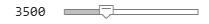3500; T_load=0.01; pmsm.I_rated=2.5; mcbPMSMCharacteristics(pmsm,inverter,'speed',w_rpm,'torque',T_load); xlim([-10.9 6.0]) ylim([-12.0 7.7]) legend("Position",[0.32518,0.11984,0.61786,0.2869])```### Case Studies

This section provides case studies that show how to find a possible control trajectory for an IPMSM and SPMSM. The case studies use the following workflow:

1. Plot the drive characteristics (including MTPV) and the constraint curves using the `mcbPMSMCharacteristics` function. For this plot, use the corner speed.

2. Use the `mcbPMSMCharacteristics` function with `driveCharacteristics` set to `0` and `opacity` set to `0.5` to plot the constraint curves over the figure generated in the previous step. For this plot, use the speed at which MTPV starts.

3. Use the `mcbPMSMCharacteristics` function with `driveCharacteristics` set to `0` and `opacity` set to `0.3` to plot the constraint curves over the figure generated in the previous step. For this plot, use the maximum speed.

Doing so plots the three voltage limiting curves overlapping the current limit circle at appropriate points in the final figures for both motors. These figures show the current trajectory for field weakening along the highlighted cyan line. This line follows the MTPV path. When the motor resistance is high enough, the MTPV curve shifts dynamically along with the speed, resulting in the current trajectory not following any single MTPV curve. The resulting cyan curve is the achievable MTPV curve because the example uses the actual equations to dynamically solve for operating points and plot the curves.

To observe the shift in the MTPV curve at different resistance values, use the drop-down menu in the following sections to pick a division factor for the resistance. With a higher resistance, the MTPV curves are farther apart, and with a lower resistance, the MTPV curves are closer to each other.

Plot these characteristics for each type of motor.

#### IPMSM Case Study

Set the field values and plot the characteristics.

```inverter.V_dc=24; pmsm.p=4; pmsm.Rs=0.45; pmsm.Rs=pmsm.Rs/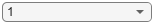1; pmsm.Ld=1e-3; pmsm.Lq=1.25*pmsm.Ld; pmsm.FluxPM=5.2e-3; pmsm.I_rated=10; pmsm.B=1.16e-5; [milestone_speeds]=mcbPMSMSpeeds(pmsm,inverter); mcbPMSMCharacteristics(pmsm,inverter,'speed',milestone_speeds(1),'driveCharacteristics',1,'constraintCurves',1)``````mcbPMSMCharacteristics(pmsm,inverter,'speed',milestone_speeds(2),'driveCharacteristics',0,'constraintCurves',1,'opacity',0.5) mcbPMSMCharacteristics(pmsm,inverter,'speed',milestone_speeds(end),'driveCharacteristics',0,'constraintCurves',1,'opacity',0.3)```#### SPMSM Case Study

Set the field values and plot the characteristics.

```inverter.V_dc=24; pmsm.p=4; pmsm.Rs=0.45; pmsm.Rs=pmsm.Rs/1; pmsm.Ld=1e-3; pmsm.Lq=pmsm.Ld; pmsm.FluxPM=5.2e-3; pmsm.I_rated=10; pmsm.B=1.16e-5; [milestone_speeds]=mcbPMSMSpeeds(pmsm,inverter); mcbPMSMCharacteristics(pmsm,inverter,'speed',milestone_speeds(1),'driveCharacteristics',1,'constraintCurves',1)``````mcbPMSMCharacteristics(pmsm,inverter,'speed',milestone_speeds(2),'driveCharacteristics',0,'constraintCurves',1,'opacity',0.5) mcbPMSMCharacteristics(pmsm,inverter,'speed',milestone_speeds(end),'driveCharacteristics',0,'constraintCurves',1,'opacity',0.3)```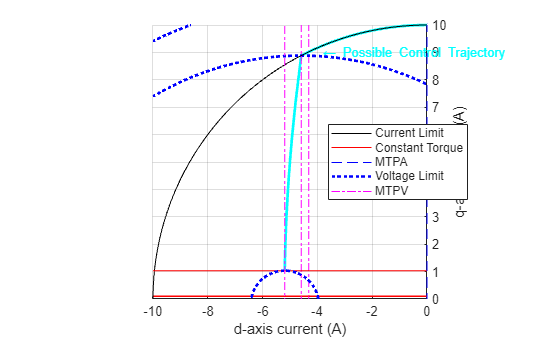Note: You can use the Tab button to get a list of supported optional arguments.

In the command line, in the live-script environment, or in the editor, the function provides suggestions for the name-value arguments.